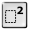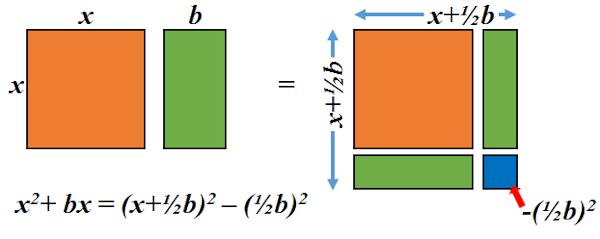# Completing the Square

## Practise this technique for use in solving quadratic equations and analysing graphs.

##### Menu  Level 1Level 2Level 3Level 4  Exam    Help    More

Write the following expressions in the completed square form.

This is level 1; Expressions with two terms such as $$x^2 + 6x$$.

 $$x^2+4x$$$$x^2+8x$$$$x^2+12x$$$$x^2+14x$$$$x^2-16x$$$$x^2+20x$$$$x^2-22x$$$$x^2+24x$$$$x^2-26x$$Check

This is Completing the Square level 1. You can also try:
Level 2 Level 3 Level 4

## Instructions

Try your best to answer the questions above. Type your answers into the boxes provided leaving no spaces. As you work through the exercise regularly click the "check" button. If you have any wrong answers, do your best to do corrections but if there is anything you don't understand, please ask your teacher for help.

When you have got all of the questions correct you may want to print out this page and paste it into your exercise book. If you keep your work in an ePortfolio you could take a screen shot of your answers and paste that into your Maths file.

## Transum.org

This web site contains over a thousand free mathematical activities for teachers and pupils. Click here to go to the main page which links to all of the resources available.## More Activities:

Mathematicians are not the people who find Maths easy; they are the people who enjoy how mystifying, puzzling and hard it is. Are you a mathematician?

Comment recorded on the 1 February 'Starter of the Day' page by Terry Shaw, Beaulieu Convent School:

"Really good site. Lots of good ideas for starters. Use it most of the time in KS3."

Comment recorded on the 7 December 'Starter of the Day' page by Cathryn Aldridge, Pells Primary:

"I use Starter of the Day as a registration and warm-up activity for my Year 6 class. The range of questioning provided is excellent as are some of the images.
I rate this site as a 5!"

Each month a newsletter is published containing details of the new additions to the Transum website and a new puzzle of the month.

The newsletter is then duplicated as a podcast which is available on the major delivery networks. You can listen to the podcast while you are commuting, exercising or relaxing.

Transum breaking news is available on Twitter @Transum and if that's not enough there is also a Transum Facebook page.

##### Featured ActivityThe Transum Newsletter for October 2023 has just been published. Click on the image above to read about the latest developments on this site and try to solve the puzzle of the month. You can read the newsletter online or listen to it by downloading the podcast.

There are answers to this exercise but they are available in this space to teachers, tutors and parents who have logged in to their Transum subscription on this computer.

A Transum subscription unlocks the answers to the online exercises, quizzes and puzzles. It also provides the teacher with access to quality external links on each of the Transum Topic pages and the facility to add to the collection themselves.

Subscribers can manage class lists, lesson plans and assessment data in the Class Admin application and have access to reports of the Transum Trophies earned by class members.

Subscribe

## Go Maths

Learning and understanding Mathematics, at every level, requires learner engagement. Mathematics is not a spectator sport. Sometimes traditional teaching fails to actively involve students. One way to address the problem is through the use of interactive activities and this web site provides many of those. The Go Maths page is an alphabetical list of free activities designed for students in Secondary/High school.

## Maths Map

Are you looking for something specific? An exercise to supplement the topic you are studying at school at the moment perhaps. Navigate using our Maths Map to find exercises, puzzles and Maths lesson starters grouped by topic.

## Teachers

If you found this activity useful don't forget to record it in your scheme of work or learning management system. The short URL, ready to be copied and pasted, is as follows:

Alternatively, if you use Google Classroom, all you have to do is click on the green icon below in order to add this activity to one of your classes.

It may be worth remembering that if Transum.org should go offline for whatever reason, there is a mirror site at Transum.info that contains most of the resources that are available here on Transum.org.

When planning to use technology in your lesson always have a plan B!

Tuesday, December 6, 2016

Do you have any comments? It is always useful to receive feedback and helps make this free resource even more useful for those learning Mathematics anywhere in the world. Click here to enter your comments.For All:

Scan the QR code below to visit the online version of this activity.https://Transum.org/go/?Num=650

## Description of Levels

CloseLevel 1 - Expressions with two terms such as $$x^2 + 6x$$

Level 2 - Expressions with three terms such as $$x^2 + 4x - 7$$

Level 3 - The coefficient of the squared term is greater than one such as $$2x^2 + 8x - 9$$

Level 4 - Use the ability to complete the square to help solve these basic quadratic equations

More Quadratic Equations - Use the ability to complete the square to help solve these more difficult quadratic equations.

Exam Style questions take the skill of completing the square and put it to use solving real problems. Typically problems involve solving equations or describing features of graphs. The questions are in the style of GCSE or IB/A-level exam paper questions and worked solutions are available for Transum subscribers.

Answers to this exercise are available lower down this page when you are logged in to your Transum account. If you don’t yet have a Transum subscription one can be very quickly set up if you are a teacher, tutor or parent.

## Curriculum Reference

See the National Curriculum page for links to related online activities and resources.

## Completing The Square

The video above is from the creative and aesthetically mindful Beth.Completing the square is a technique used to manipulate quadratic expressions into a standard form, which allows for easier factorisation or solution finding.

For example, to complete the square for the quadratic expression $$x^2 + 6x + 5$$, we follow these steps:

\begin{aligned} x^2 + 6x + 5 &= (x + 3)^2 - 9 + 5 \\ &= (x + 3)^2 - 4 \end{aligned}

Therefore, the quadratic expression $$x^2 + 6x + 5$$ can be written in the standard form $$(x + 3)^2 - 4$$ after completing the square.

The key formula to complete the square for a quadratic expression of the form $$ax^2 + bx + c$$ is:

$$ax^2 + bx + c = a\left(x + \frac{b}{2a}\right)^2 - \frac{b^2}{4a} + c$$

where $$a, b,$$ and $$c$$ are constants.

Completing the square is a useful technique in solving quadratic equations and graphing quadratic functions, among other applications.

Don't wait until you have finished the exercise before you click on the 'Check' button. Click it often as you work through the questions to see if you are answering them correctly.

Answers to this exercise are available lower down this page when you are logged in to your Transum account. If you don’t yet have a Transum subscription one can be very quickly set up if you are a teacher, tutor or parent.

Close